11 篇文章 2 订阅

1、平滑法
2、趋势拟合法
3、组合模型
4、AR模型
5、MA模型
6、ARMA模型
7、ARIMA模型
8、ARCH模型
9、GARCH模型及其衍生模型

# 平滑法

## 2、移动平均法

### 2.1、简单移动平均

由于算术平均数、几何平均数、调和平均数、加权算术平均数的计算方法相对其余几种来说，比较简单，故常称这几种平均数的求法为“简单平均法”。

F ( t + 1 ) = V ( 1 ) + V ( 2 ) + . . . + V ( t ) t (1) F(t + 1) = \frac{V(1)+V(2)+...+V(t)}{t}\tag{1}

## 2.2、加权移动平均法

F n + 1 = ∑ i = n − k − 1 n + 1 V i x i (2) F_{n+1}= \sum_{i=n-k-1}^{n+1}V_{i}x_{i}\tag{2}

----------------------------------------------------------------------------------------------------------------------
例题：某商场1月份至11月份的实际销售额如表所示。假定跨越期为3个月，权数为l、2、3，试用加权移动平均法预测12月份的销售额

138-
245-
33538.83
44943.67
57043.67
64357.17
74653.00
85549.00
94550.00
106848.5
116458.17
1262.17

V 4 = 3 ∗ 35 + 2 ∗ 45 + 1 ∗ 38 1 + 2 + 3 = 38.83 V 5 = 3 ∗ 49 + 2 ∗ 35 + 1 ∗ 45 1 + 2 + 3 = 43.67 … … F ( 12 ) = 3 ∗ 64 + 2 ∗ 68 + 1 ∗ 45 1 + 2 + 3 = 62.17 V_{4}=\frac{3*35 + 2*45 +1*38}{1+2+3}=38.83\\ V_{5}=\frac{3*49 + 2*35 +1*45}{1+2+3}=43.67\\ ……\\ \\ \\ F(12)=\frac{3*64 + 2*68 +1*45}{1+2+3}=62.17

----------------------------------------------------------------------------------------------------------------------

## 3、指数平滑法

### 3.1、一次指数平滑

S t ( 1 ) = α ⋅ y t + ( 1 − α ) ⋅ S t − 1 ( 1 ) (3) S_{t}^{(1)}=\alpha \cdot y_{t} +( 1- \alpha)\cdot S_{t-1}^{(1)} \tag{3}

• S t S_{t} 具有逐期追溯的性质，可包括全部数据，但实际计算时，仅需要两个值，即 y t y_{t} S t − 1 ( 1 ) S_{t-1}^{(1)} ;
• 平滑指数 α \alpha 以指数形式递减，故称之为指数平滑法。平滑指数取值很重要，平滑常数 α \alpha 决定了平滑水平以及对预测值与实际结果之间差异的响应速度： α \alpha 越接近于1，远期实际值对本期平滑值影响程度下降的越快；越接近于0，远期实际值对本期平滑值的影响程度下降的越慢。所以，当时间数列相对平稳时，可取较大 α \alpha ；当时间数列波动较大时，应取较小的 α \alpha ，以此不忽略远期实际值的影响。经验如下（他人）：指数平滑系数α的确定
（1）当时间序列呈现较稳定的水平趋势时，应选较小的α，一般可在0.05~0.20之间取值‘
（2）当时间序列有波动，但长期趋势变化不大时，可选稍大的α值，常在0.1~0.4之间取值；
（3）当时间序列波动很大，长期趋势变化幅度较大，呈现明显且迅速的上升或下降趋势时，宜选择较大的α值，如可在0.6~0.8间选值。以使预测模型灵敏度高些，能迅速跟上数据的变化。
（4）当时间序列数据是上升（或下降）的发展趋势类型，α应取较大的值，在0.6~1之间。

（1）如果能够找到 y 1 y_{1} 以前的历史资料，那么当数据较少时，使用全期平均、移动平均；当数据较多时，可用最小二乘法。
（2）如果仅有从 y 1 y_{1} 开始的数据，那么确定初始值的方法：取 S 1 ( 1 ) = y 1 S_{1}^{(1)}=y_{1} ;待积累若干数据后，取 S 1 S_{1} 等于前面若干数据的简单算数平均数，如： S 0 ( 1 ) = y 1 + y 2 + y 3 3 S_{0}^{(1)}=\frac{y_{1}+y_{2}+y_{3}}{3}
-------------------------------------------------------------------------------------------------------------------------------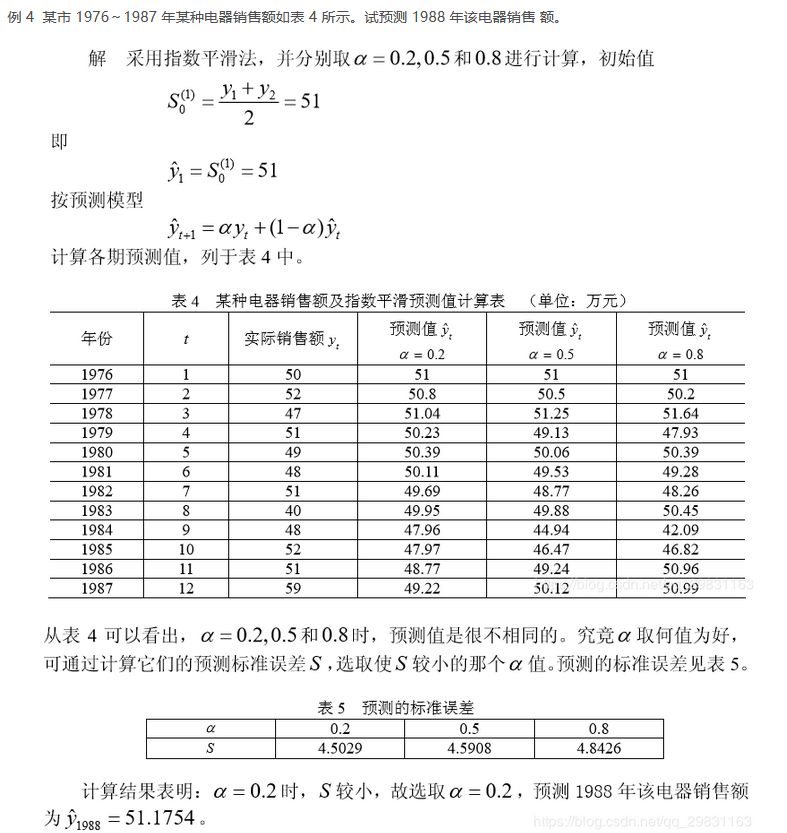### 3.2 二次平滑指数

S t ( 1 ) = α y t + ( 1 − α ) S t − 1 ( 1 ) S t ( 2 ) = α S t ( 1 ) + ( 1 − α ) S t − 1 ( 2 ) (4) S_{t}^{(1)}=\alpha y_{t}+(1-\alpha)S_{t-1}^{(1)}\\ S_{t}^{(2)}=\alpha S_{t}^{(1)}+(1-\alpha)S_{t-1}^{(2)} \tag{4}

y ^ t + T = a t + b t T (5) \hat{y}_{t+T}= a_{t}+b_{t}T\tag{5}

{ a t = 2 S t ( 1 ) − S t ( 2 ) b t = α 1 − α ( S t ( 1 ) − S t ( 2 ) ) (6) \left\{\begin{matrix} a_{t}=2S_{t}^{(1)}-S^{(2)}_{t}\\ b_{t}=\frac{\alpha}{1-\alpha}(S^{(1)}_{t}-S^{(2)}_{t}) \end{matrix}\right.\tag{6}
-------------------------------------------------------------------------------------------------------------------------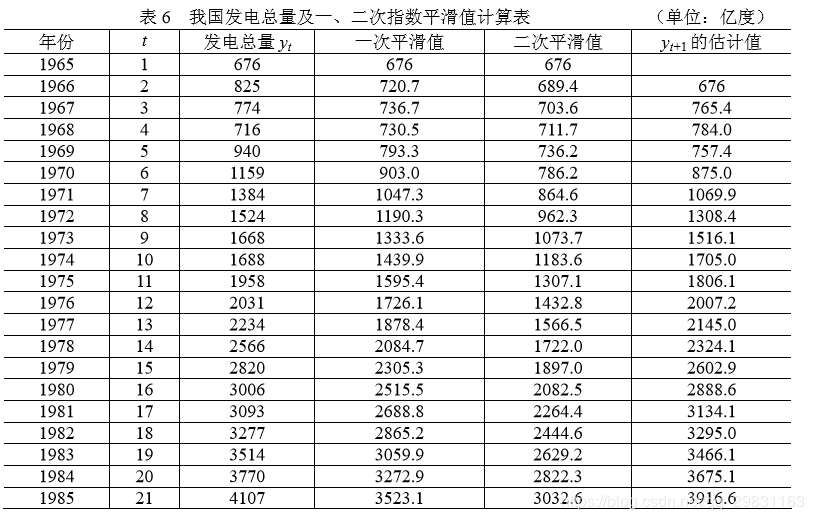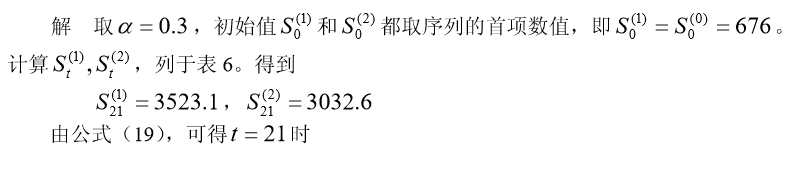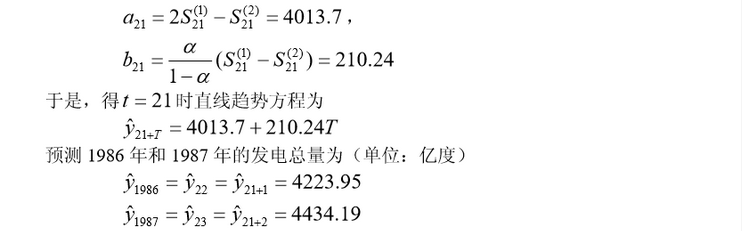为了求各期的模拟值，可将式(6)带入直线模型(5)，并另T = 1,则得：
y ^ t + 1 = ( 2 S t ( 1 ) − S t ( 2 ) ) + α 1 + α ( S t ( 1 ) − S t ( 2 ) ) (7) \hat{y}_{t+1}=(2S_{t}^{(1)}-S_{t}^{(2)})+\frac{\alpha}{1+\alpha}(S_{t}^{(1)}-S_{t}^{(2)})\tag{7}

y ^ t + 1 = ( 1 + 1 1 + α ) S t ( 1 ) − 1 1 − α S t ( 2 ) (8) \hat{y}_{t+1}=(1+\frac{1}{1+\alpha})S_{t}^{(1)}-\frac{1}{1-\alpha}S_{t}^{(2)}\tag{8}

### 3.3、三次平滑指数

{ S t ( 1 ) = α y t + ( 1 + α ) S t ( 1 ) S t ( 2 ) = α S t ( 1 ) + ( 1 + α ) S t − 1 ( 2 ) S t ( 3 ) = α S t ( 2 ) + ( 1 + α ) S t − 1 ( 3 ) (9) \left\{\begin{matrix} S_{t}^{(1)}=\alpha y_{t}+(1 +\alpha )S_{t}^{(1)}\\S_{t}^{(2)}=\alpha S_{t}^{(1)}+(1 +\alpha )S_{t-1}^{(2)}\\S_{t}^{(3)}=\alpha S_{t}^{(2)}+(1 +\alpha )S_{t-1}^{(3)} \end{matrix}\right.\tag{9}

y ^ = a t + b t T + C t T 2 (10) \hat{y}=a_{t}+b_{t}T+C_{t}T^{2}\tag{10}

{ a t = 3 S t ( 1 ) − 3 S t ( 2 ) + S t ( 3 ) b t = α 2 ( 1 + α ) [ ( 6 − 5 α ) S t ( 1 ) − 2 ( 5 − 4 α ) S t ( 2 ) + ( 4 − 3 α ) S t ( 3 ) ] c t = α 2 2 ( 1 − α ) 2 [ S t ( 1 ) − 2 S t ( 2 ) + S t ( 3 ) ] (11) \left\{\begin{matrix} a_{t}=3S_{t}^{(1)}-3S_{t}^{(2)}+S_{t}^{(3)}\\b_{t}=\frac{\alpha}{2(1+\alpha)}[(6-5\alpha)S_{t}^{(1)}-2(5-4\alpha)S_{t}^{(2)}+(4-3\alpha)S_{t}^{(3)}]\\c_{t}=\frac{\alpha ^{2}}{2(1-\alpha)^{2}}[S_{t}^{(1)}-2S_{t}^{(2)}+S_{t}^{(3)}] \end{matrix}\right.\tag{11}
----------------------------------------------------------------------------------------------------------------------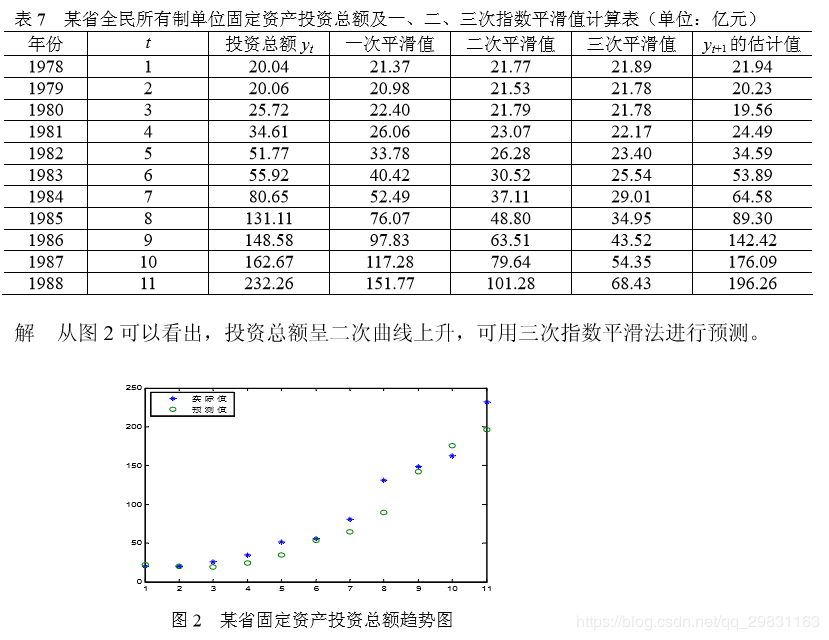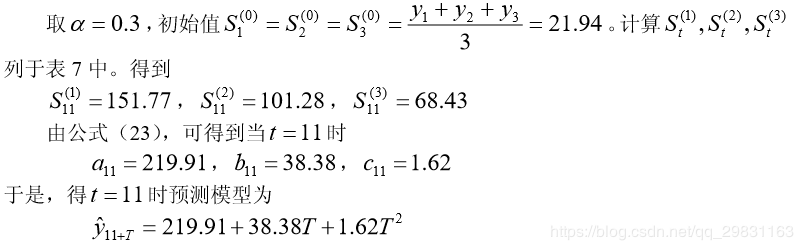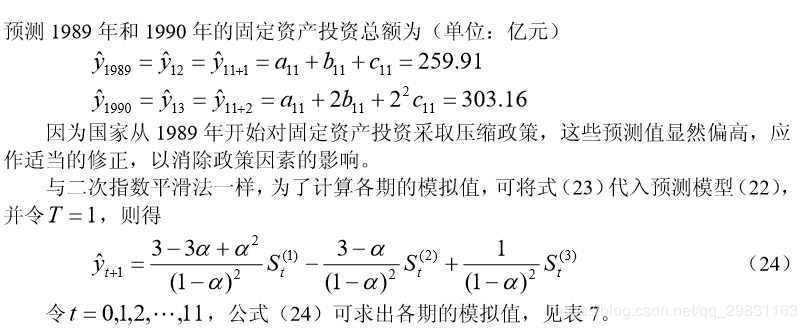01-04
04-221万+09-121349
09-178674
12-236561
11-135万+
11-071499
04-212万+
07-303586
04-145万+
05-081万+
09-061076
12-121142
04-012916
10-256万+
09-075万+
07-2111万+
08-187178
07-271万+
06-051774点击重新获取扫码支付余额充值